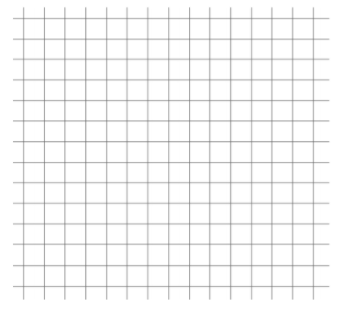# Linear Relationships

## Objective

Model real-world situations with linear relationships.

## Common Core Standards

### Core Standards

?

• 8.F.B.4 — Construct a function to model a linear relationship between two quantities. Determine the rate of change and initial value of the function from a description of a relationship or from two (x, y) values, including reading these from a table or from a graph. Interpret the rate of change and initial value of a linear function in terms of the situation it models, and in terms of its graph or a table of values.

?

• 7.EE.B.4

## Criteria for Success

?

1. Write and graph equations to model real-world situations with linear relationships (MP.4).
2. Interpret the slope of the line and the $y-$intercept in context of the situation (MP.2).

## Tips for Teachers

?

In this lesson, students bring several concepts and skills together to be able to model and interpret real-world linear situations. The Problem Set Guidance includes several resources that can be used in connection to this lesson or as part of a review for the unit.

### Remote Learning Guidance

If you need to adapt or shorten this lesson for remote learning, we suggest prioritizing Anchor Problem 1 or 2 (benefit from discussion) . Find more guidance on adapting our math curriculum for remote learning here.

#### Fishtank Plus

Subscribe to Fishtank Plus to unlock access to additional resources for this lesson, including:

• Problem Set
• Student Handout Editor
• Google Classrom Integration
• Vocabulary Package

## Anchor Problems

?

### Problem 1

A student has had a collection of baseball cards for several years. Suppose that B, the number of cards in the collection, can be described as a function of $t$, which is time in years since the collection was started.

Explain what each of the following equations would tell us about the number of cards in the collection over time.

1.   $B=200+100t$
2.   $B=100+200t$
3.   $B=2000-100t$
4.   $B=100-200t$

#### Guiding Questions

Create a free account or sign in to access the Guiding Questions for this Anchor Problem.

#### References

Illustrative Mathematics Baseball Cards

Baseball Cards, accessed on Dec. 6, 2016, 3:22 p.m., is licensed by Illustrative Mathematics under either the CC BY 4.0 or CC BY-NC-SA 4.0. For further information, contact Illustrative Mathematics.

### Problem 2

You have $100 to spend on a barbeque where you want to serve chicken and steak. Chicken costs$1.30 per pound and steak costs 3.50 per pound. You want to know how many pounds of chicken and steak you can afford to buy. 1. Write and graph an equation that relates the amount of chicken and the amount of steak you can buy. 2. What is the meaning of each intercept in this context? 3. What is the meaning of the slope in this context? 4. Discuss what your options are for the amounts of chicken and steak you can buy for the barbeque. #### Guiding Questions Create a free account or sign in to access the Guiding Questions for this Anchor Problem. #### References Illustrative Mathematics Chicken and Steak, Variation 1 Chicken and Steak, Variation 1, accessed on Feb. 26, 2018, 11:31 a.m., is licensed by Illustrative Mathematics under either the CC BY 4.0 or CC BY-NC-SA 4.0. For further information, contact Illustrative Mathematics. Modified by Fishtank Learning, Inc. ## Problem Set ?With Fishtank Plus, you can download a complete problem set and answer key for this lesson. Download Sample The following resources include problems and activities aligned to the objective of the lesson that can be used to create your own problem set. ## Target Task ? A parking garage is located in the downtown area of a city. The table below shows the cost for parking in the garage for different amounts of time.  Hours Parked Cost of Parking 18.80 $1 \frac{1}{2}$ $10.70 4$20.20 5 $24 $7 \frac{1}{2}$$33.50 10 \$43

a.   What equation represents the cost of parking in the garage, $y$, for $x$ hours?

b.   Sketch a graph to represent the cost of parking over time.c.   What does the slope of your line represent in context of this situation?

d.   What does the $y$-intercept of your line represent in context of this situation?

#### Mastery Response

Create a free account or sign in to view Mastery Response